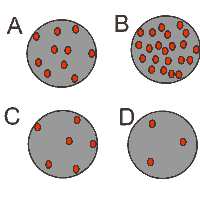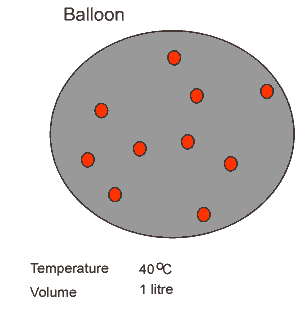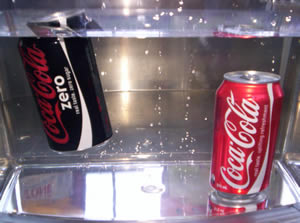Density

Density is the mass of a substance per unit of volume. Density depends on the number and mass of particles that make up the substance in a given volume of the substance.

The image on the right shows the number of particles of a substance at four different stages. The volume of the substance is fixed. Stage "B" represents the stage with the greatest density while stage "D" is the least dense.The density of a substance can be increased by decreasing its volume. As warm air cools, its volume decreases and its density increases. When a balloon with warm air is cooled its volume decreases and the air inside increases in density. This is shown in the animation on the left.

If we know the mass of a substance and its volume we can calculate its density using the expression

Density = mass/volume

Design an experiment to calculate the density of different fruit. Click to see a hint on how you can measure the volume of the fruit.

 Fruit Mass(grams) Volume Density apple banana orange pear plumb

Density of rocks -activity

Exercises

Continue

Assuming that each can contains 375 mL of liquid what can you say about the density of Coke Zero as compared to normal Coke?

Each can contains 375 mL of liquid. Normal Coke contains 40.0 g of sugar in each can while Zero contains 0.4 grams of sugar. Calculate the density of each liquid assuming it contains only sugar and water.

If the density of water is 1.0g/cm3 explain why Coke Zero floats while normal Coke sinks.